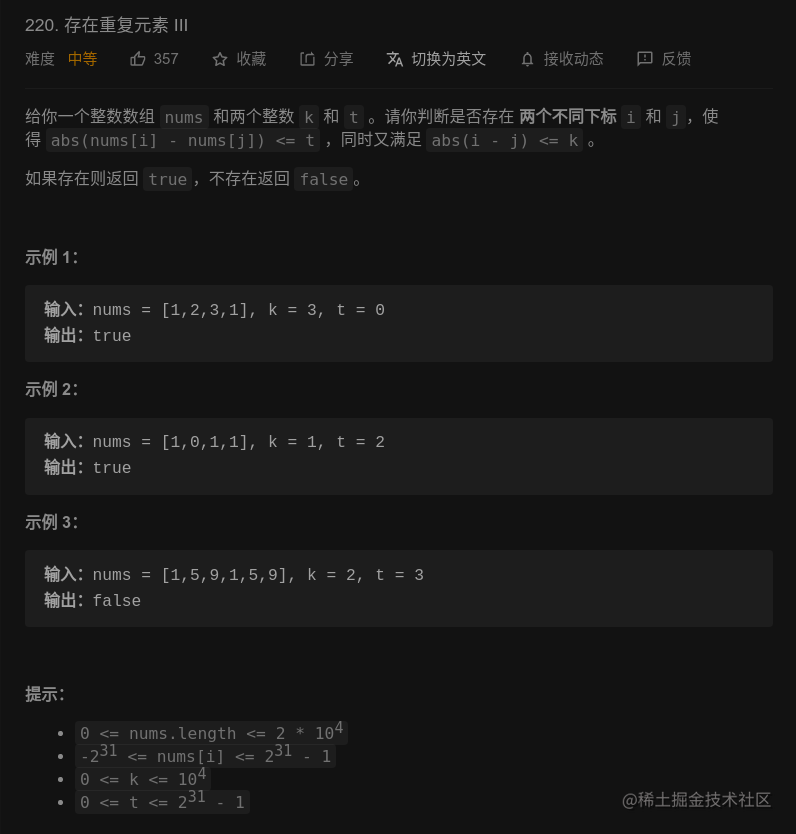# LeetCode 220. 存在重复元素 III：题解｜刷题打卡

## 一、题目描述：## 二、思路分析：

1. 因为两个元素的下标 $i$$j$ 需要满足 $abs(i - j) \leq k$，所以我们可以在遍历到元素 $x$ 的时候只考虑在它前面的 $k$ 个元素里查找是否存在满足条件的元素，但是一般的数据结构查找的效率较低，我们可以使用有序集合 $set$ 记录滑动窗口里的元素，再通过二分查找来优化查找的效率。

2. 虽然数组里可能存在重复元素，但是很容易可以证明只要滑动窗口遇到了重复元素，通过查找就会直接返回 $true$，所以可以直接使用 $set$ 而无需 $multiset$

3. 使用有序集合的解法比较直观，建议读者自己尝试，我在这里想讨论的主要是使用桶的做法。前面提到一般的数据结构查找元素的效率较低，有序集合的二分查找复杂度是 $O(log(n))$，那有没有查找时间更优的数据结构呢？当然有，答案就是桶。

4. 对于元素 $x$ 来说，满足条件的元素属于 $[x - t, x + t]$，这个区间的长度是 $2t + 1$，如果我们把整个 $int$ 范围的数都都按照 $t + 1$ 的长度划分到不同的桶里，那么很容易就可以推出：假如 $x$ 属于 $id$ 号桶，那么 $id$ 号桶还有其他元素就说明满足条件，如果 $id$ 号桶没有，那么我们只需要再判断相邻的两个桶有没有与 $x$ 的差值小于等于 $t$ 的元素即可。

5. 因为只要有一个桶包含超过一个元素就可以返回 $true$，所以我们可以直接使用哈希表来实现桶。一个需要注意的细节是因为数据范围为 $int$ 范围，所以在进行加减法的时候需要小心溢出。

6. 分桶的时候如果直接令桶 $id = \lfloor x / (t + 1)\rfloor$，会导致 $[-t + 1, t - 1]$ 都属于第 $0$ 组，不满足按长度 $t + 1$ 分桶的原则，解决的办法是对 $x < 0$ 的情况进行特殊处理让 $[-t - 1, -1]$ 的元素归于 $-1$ 桶，更小的元素按照长度 $t + 1$ 依次划分。

## 三、AC 代码：

class Solution {
public:
int getId(int x, long w) {
// 对负数进行特殊处理可以防止 0 既属于 0 组，也属于 -1 组
// 从而使每个 ID 所涵盖的元素个数均为 w 个
return x < 0 ? (x + 1ll) / w - 1 : x / w;
}
bool containsNearbyAlmostDuplicate(vector<int>& nums, int k, int t) {
unordered_map<int, int> mp;
int n = nums.size();
for (int i = 0; i < n; ++i) {
// 防止后续的减操作导致溢出
long x = nums[i];
int id = getId(x, t + 1ll);
if (mp.count(id)) {
return true;
}
if (mp.count(id - 1) && x - mp[id - 1] <= t) {
return true;
}
if (mp.count(id + 1) && mp[id + 1] - x <= t) {
return true;
}
mp[id] = x;
if (i >= k) {
mp.erase(getId(nums[i - k], t + 1ll));
}
}
return false;
}
};